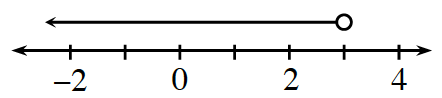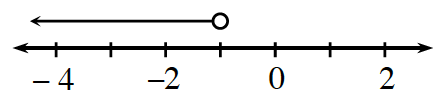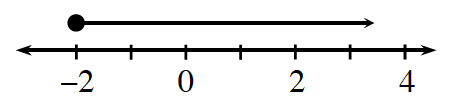### Home > CC2 > Chapter 7 > Lesson 7.1.5 > Problem7-60

7-60.

Write the inequality represented by each graph.  Homework Help ✎

1.Since the arrow is pointing to the left, that means that all those values are less than the selected point.

Therefore, any value to the left of $3$ is less than $3$. Since the circle above the selected point is open, the point itself is not included and you need to use either $<$ or $>$.

$x<3$

1.See part (a).

1.Notice that this time the circle above the selected point is shaded in, so the point itself is included. That means you will use either $\le$ or $\ge$. Since the arrow is pointing to the right, that means that all those values are greater than the selected point.